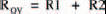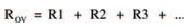# The second circuitElectronics guide > On the boards > The second circuit

Figure 2.4 shows the next circuit we’re going to look at and how to build it on breadboard. It’s really just another simple circuit, this time consisting of two resistors in a line — we say they’re in series. The aim of this experiment is to measure the overall resistance of the series resistors and see if we can devise a formula which allows us to calculate other series resistors’ overall resistances without the need of measurement.Figure 2.4 Two resistors mounted on the breadboard in series

Figure 2.5 shows the more usual way of representing a circuit in a drawing — the circuit diagram. What we have done is replace the actual resistor shapes with symbols. Resistor symbols are zig-zag lines usually, although sometimes small oblong boxes are used in circuit diagrams. The resistors in the circuit diagram are numbered R1 and R2, and their values are shown, too.Figure 2.5 A circuit diagram of two resistors in series. The meter is represented by a round symbol

Meters in circuit diagrams are shown as a circular symbol, with an arrow to indicate the pointer. To show it’s a resistance multi-meter (that is, an ohm-multi-meter, more commonly called just ohmmeter) the letter R is shown inside it. While we’re on the topic of circuit diagram symbols, Figure 2.6 shows a few very common ones (including resistor and meter) which we’ll use in this book. Look out for them later!

You should have noticed that there is no indication of the breadboard in the circuit diagram of Figure 2.5. There is no need. The circuit diagram is merely a way of showing components and their electrical connections. The physical connection details are in the breadboard layout diagram of Figure 2.4. From now on, we’ll be using two such diagrams with every new circuit. If you’re feeling particularly adventurous you might care to build your own circuit on breadboard, following only the circuit diagram — not the associated breadboard layout. It doesn’t matter if your circuit has a different layout to ours, it will still work as long as all the electrical connections are there.Figure 2.6 Commonly used symbols

Back to the circuit: it’s now time to measure the overall resistance of the series resistors. Following the same instructions we gave you before, do it!

If your measurement is correct you should have a reading of 20 k. But what does this prove? Well, it suggests that there is a relationship between the separate resistors (each of value 10 k) and the overall resistance. It looks very much as though the overall resistance (which we call, say, ROV) equals R1 + R2. Or put mathematically:But how can we test this? The easiest way is to change the resistors. Try doing the experiment with two different resistors. You’ll find the same is true: the overall resistance always equals the sum of the two separate resistances.

By experiment, we’ve just proved the law of series resistors. And it doesn’t just stop at two resistors in series. Three, four, five, in fact, any number of resistors may be in series — the overall resistance is the sum of the individual ones. This can be summarised mathematically as:Try it yourself!

<< Experiment